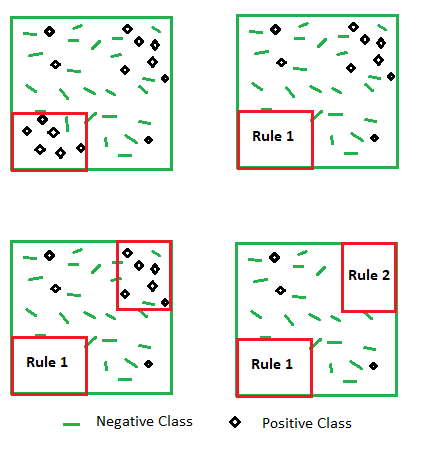# Rule-Based Classifier – Machine Learning

• Difficulty Level : Medium
• Last Updated : 12 Jan, 2022

Rule-based classifiers are just another type of classifier which makes the class decision depending by using various “if..else” rules. These rules are easily interpretable and thus these classifiers are generally used to generate descriptive models. The condition used with “if” is called the antecedent and the predicted class of each rule is called the consequent

Properties of rule-based classifiers:

• Coverage: The percentage of records which satisfy the antecedent conditions of a particular rule.
• The rules generated by the rule-based classifiers are generally not mutually exclusive, i.e. many rules can cover the same record.
• The rules generated by the rule-based classifiers may not be exhaustive, i.e. there may be some records which are not covered by any of the rules.
• The decision boundaries created by them is linear, but these can be much more complex than the decision tree because the many rules are triggered for the same record.

An obvious question, which comes into the mind after knowing that the rules are not mutually exclusive is that how would the class be decided in case different rules with different consequent cover the record.

There are two solutions to the above problem:

• Either rules can be ordered, i.e. the class corresponding to the highest priority rule triggered is taken as the final class.
• Otherwise, we can assign votes for each class depending on some their weights, i.e. the rules remain unordered.

Example:
Below is the dataset to classify mushrooms as edible or poisonous:

```Rules:

Odour = pungent and habitat = urban -> Class = poisonous
Bruises = yes -> Class = edible : This rules covers both negative and positive records.

The given rules are not mutually exclusive.```

How to generate a rule:Sequential Rule Generation.

Rules can be generated either using general-to-specific approach or specific-to-general approach. In the general-to-specific approach, start with a rule with no antecedent and keep on adding conditions to it till we see major improvements in our evaluation metrics. While for the other we keep on removing the conditions from a rule covering a very specific case. The evaluation metric can be accuracy, information gain, likelihood ratio etc.

Algorithm for generating the model incrementally:

The algorithm given below generates a model with unordered rules and ordered classes, i.e. we can decide which class to give priority while generating the rules.

A <-Set of attributes
T <-Set of training records
Y <-Set of classes
Y’ <-Ordered Y according to relevance
R <-Set of rules generated, initially to an empty list
for each class y in Y’
while the majority of class y records are not covered
generate a new rule for class y, using methods given above
Add this rule to R
Remove the records covered by this rule from T
end while
end for
Add rule {}->y’ where y’ is the default class

Classifying a record:
The classification algorithm described below assumes that the rules are unordered and the classes are weighted.

R <-Set of rules generated using training Set
T <-Test Record
W <-class name to Weight mapping, predefined, given as input
F <-class name to Vote mapping, generated for each test record, to be calculated
for each rule r in R
check if r covers T
if so then add W of predicted_class to F of predicted_class
end for
Output the class with the highest calculated vote in F

Note: The rule set can be also created indirectly by pruning(simplifying) other already generated models like a decision tree.

My Personal Notes arrow_drop_up